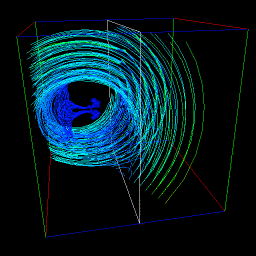# Mathematical Recreations

Last update: 15-Jun-1997

Even before there were computer graphics, I enjoyed playing with mathematical puzzles and games. The first vector was drawn by computer in the early sixties; shortly before the hidden line problem was solved in the early seventies, I began playing with using computers to illuminate mathematics. I've never been able to stop.

## Polygonal Letters

I created the letters of this polygonal alphabet in 1984 to use to test rendering hardware, and I've been carrying them around ever since.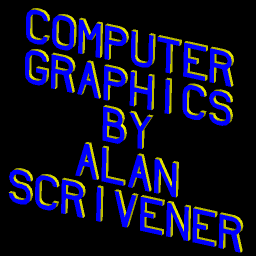## Torus Surfaces

These are algorithmically simple, yet very beautiful in my opinion.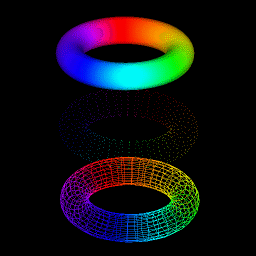## Moire Pattern

Moire patterns fascinate me, and I simulate them on every graphics system I program. What once took a weekend to compute on an Apple II can now go at real-time frame rates on an SGI Indogo 2.

```v = sin(sqrt(xc1 - x)^2 + (yc1 - y)^2)
+ sin(sqrt(xc2 - x)^2 + (yc2 - y)^2)
```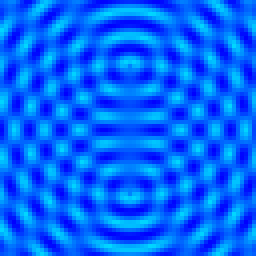## Spherical Lissajous

This was a lunch-hour hack in 1988, inspired by an article in Scientific American.

```x = R*sin(A*t)*cos(B*t)
y = R*sin(A*t)*sin(B*t)
z = R*cos(A*t)
```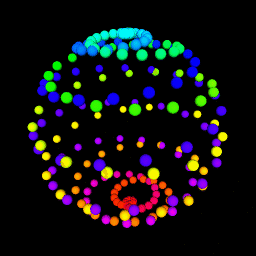## 4-D Hypercube Data Mapped Onto 3-D Tesseract

This is an illustration for a paper I have submitted for SIGGRAPH '96 in New Orleans. (See http://siggraph.org/ for more information on the conference.) The picture is a 4-D visualization of a light cone (from Einstein's Special Relativity Theory) mapped onto a tesseract (unfolded hypercube).

```sqrt(x^2 + y^2 + z^2 - t^2) = K

```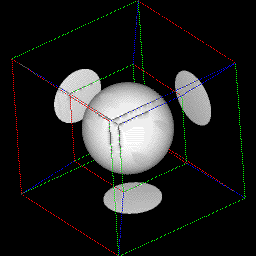# Dynamical Systems:

Since college I have been interested in systems theory, and how dynamical systems evolve by the "change rules" of Ordinary Differential Equations (ODEs). By mapping a 2-D or 3-D state space into a graphical representation, it is is possible to see many possible system behaviors at once, each represented by a trajectory through the space. Here are some systems I have visualized this way:

## Random State Space

The change vector at each point in the volume is randomly assigned.

```xdot = rand(x, y, z)
ydot = rand(x, y, z)
zdot = rand(x, y, z)
```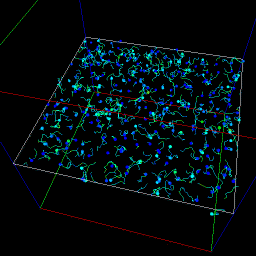## Huygens Damped Pendulum

A simple 2-D system that loses energy as it oscillates, seeking one of two stable equilibrium points. (A third unstable equilibrium point sits beteen them.)

```xdot = y
ydor = sin(x) - y
```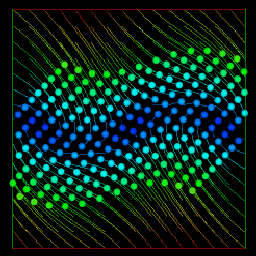## Van Der Pol Forced Oscillator

A more complex 3-D system that finds an oscillating equilibrium.

```xdot = y
ydot = -(x + y^3 - y) + cos(z)
zdot = K

K = 8
```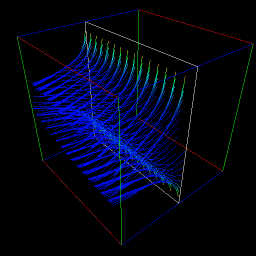## Richardson Arm's Race

A 2-D simulation of two nations in an escalating arms race, each responding to the other's arms buildup.

```xdot = -A*x + B*y + C
ydot =  D*x - E*y + F

A = B = C = D = E = F = 0.5
```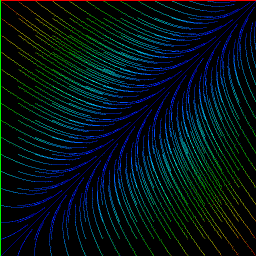## Lorenz Attractor

The famous 3-D system of thermal convection that exhibited some of the first chaos ever recognized in a mathematical model.

```xdot = A*(y - x)
ydot = B*x - y - (x*z)
zdot = x*y - C*z

A = 10
B = 28
C = 5/3
```